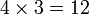# Semidirect product of Z3 and D8 with action kernel V4

This article is about a particular group, i.e., a group unique upto isomorphism. View specific information (such as linear representation theory, subgroup structure) about this group
View a complete list of particular groups (this is a very huge list!)[SHOW MORE]

## Definition

This group is defined as follow: it is the external semidirect product of cyclic group:Z3 by dihedral group:D8 where the action of the latter on the former is given by a homomorphism$D_8 \to \operatorname{Aut}(\mathbb{Z}_3)$ whose kernel is one of the Klein four-subgroups of dihedral group:D8. Note that the homomorphism is completely specified by its kernel because there is a unique isomorphism between any two groups isomorphic to cyclic group:Z2.

## Arithmetic functions

Want to compare and contrast arithmetic function values with other groups of the same order? Check out groups of order 24#Arithmetic functions
Function Value Similar groups Explanation
order (number of elements, equivalently, cardinality or size of underlying set) 24 groups with same order order of semidirect product is product of orders:$|\mathbb{Z}_3||D_8| = 3 \cdot 8 = 24$
exponent of a group 12 groups with same order and exponent of a group | groups with same exponent of a group exponent of a finite group equals product of exponents of its Sylow subgroups: In this case, by construction, the Sylow subgroups are$D_8$ and$\mathbb{Z}_3$, so the exponent is the product of their exponent, which is$4 \times 3 = 12$.
nilpotency class not a nilpotent group
derived length 2 groups with same order and derived length | groups with same derived length The derived subgroup of the acting group$D_8$ is in the kernel of the action and is abelian, and so is the base group$\mathbb{Z}_3$.
Fitting length 2 groups with same order and Fitting length | groups with same Fitting length
Frattini length 2 groups with same order and Frattini length | groups with same Frattini length
minimum size of generating set 2 groups with same order and minimum size of generating set | groups with same minimum size of generating set

## GAP implementation

### Group ID

This finite group has order 24 and has ID 8 among the groups of order 24 in GAP's SmallGroup library. For context, there are 15 groups of order 24. It can thus be defined using GAP's SmallGroup function as:

SmallGroup(24,8)

For instance, we can use the following assignment in GAP to create the group and name it$G$:

gap> G := SmallGroup(24,8);

Conversely, to check whether a given group$G$ is in fact the group we want, we can use GAP's IdGroup function:

IdGroup(G) = [24,8]

or just do:

IdGroup(G)

to have GAP output the group ID, that we can then compare to what we want.